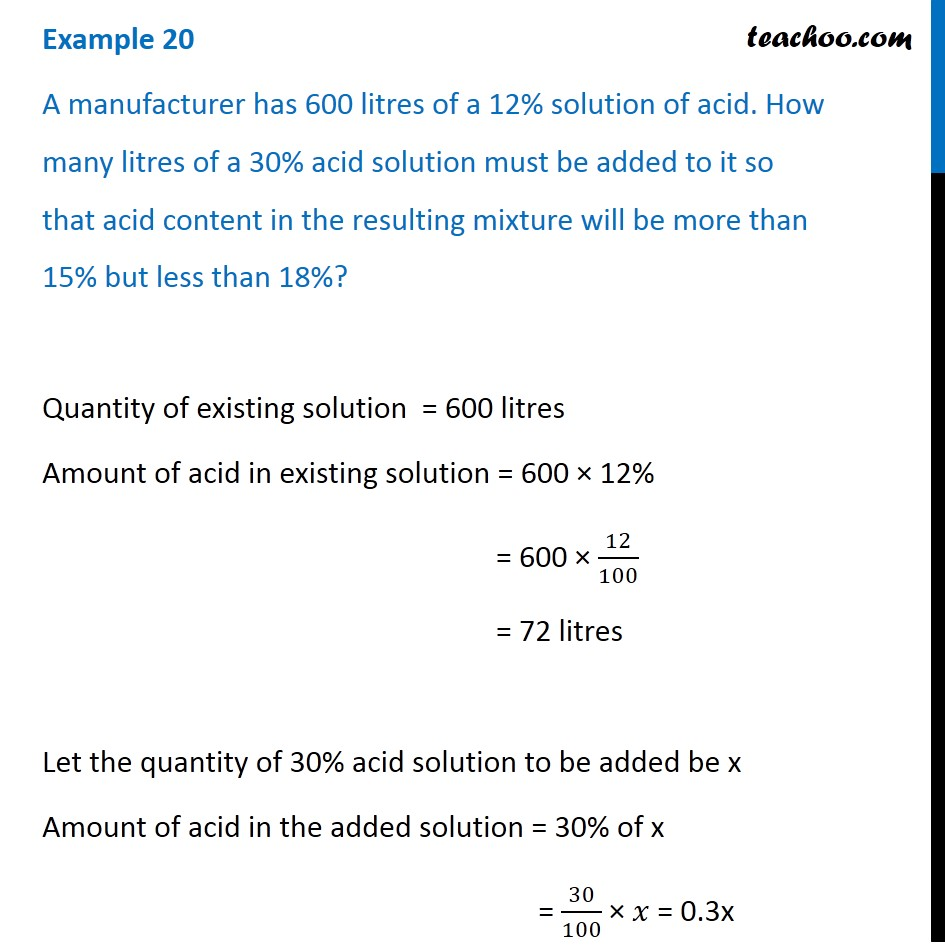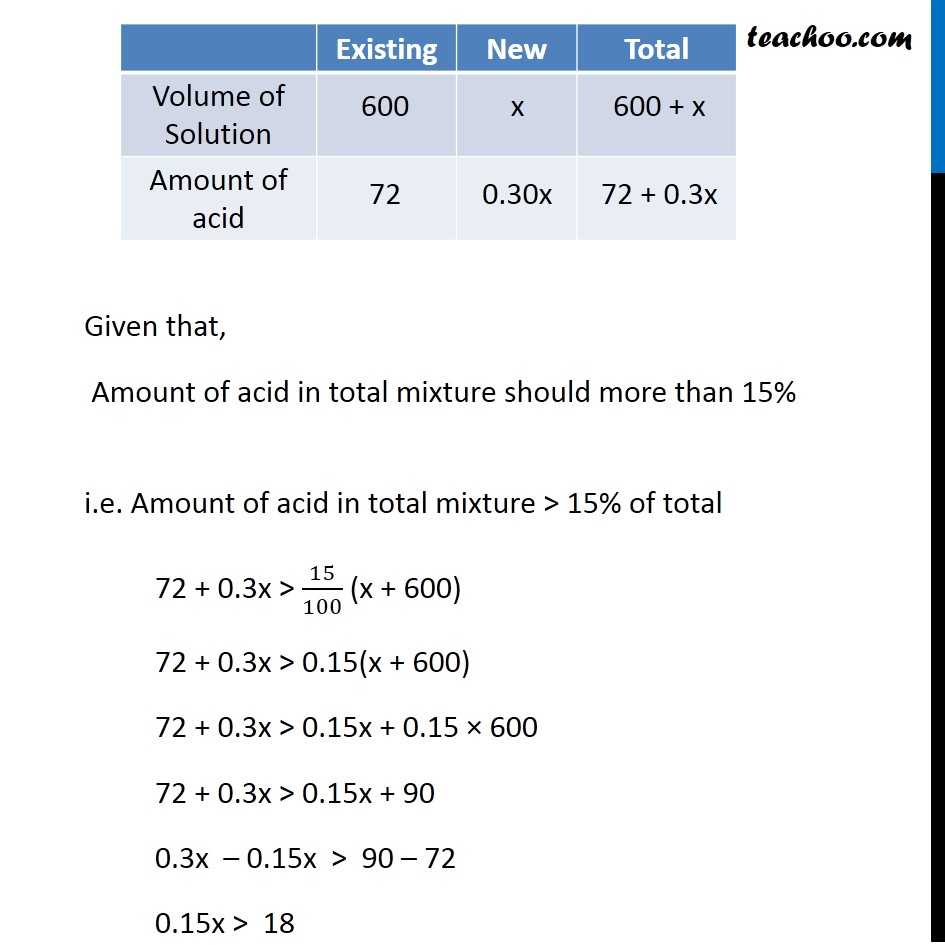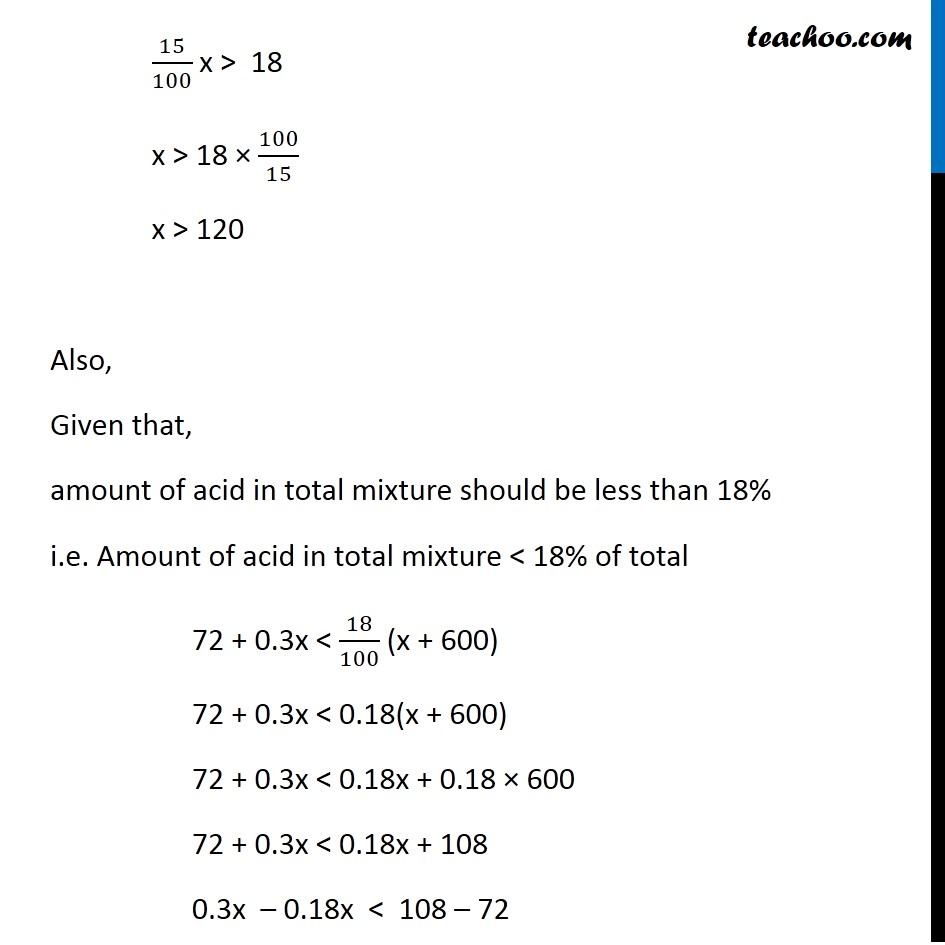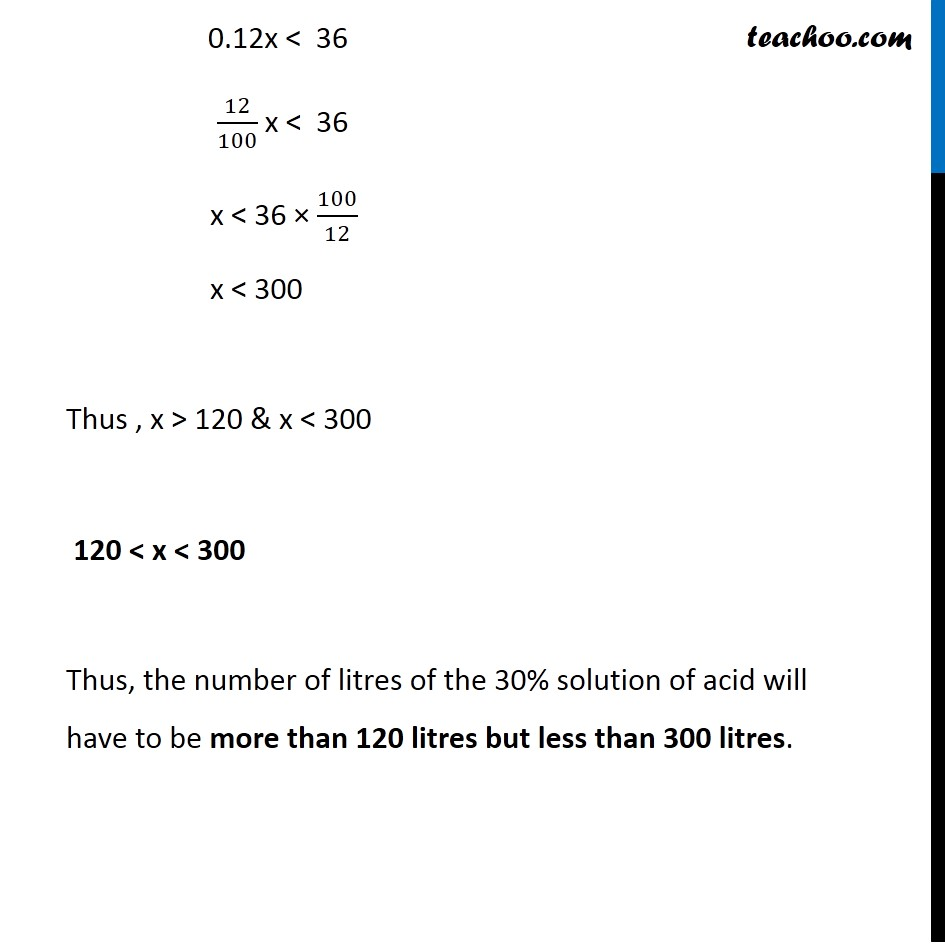Examples

Chapter 5 Class 11 Linear Inequalities
Serial order wiseLearn in your speed, with individual attention - Teachoo Maths 1-on-1 Class

### Transcript

Example 13 A manufacturer has 600 litres of a 12% solution of acid. How many litres of a 30% acid solution must be added to it so that acid content in the resulting mixture will be more than 15% but less than 18%? Quantity of existing solution = 600 litres Amount of acid in existing solution = 600 × 12% = 600 × 12/100 = 72 litres Let the quantity of 30% acid solution to be added be x Amount of acid in the added solution = 30% of x = 30/100 × 𝑥 = 0.3x Given that, Amount of acid in total mixture should more than 15% i.e. Amount of acid in total mixture > 15% of total 72 + 0.3x > 15/100 (x + 600) 72 + 0.3x > 0.15(x + 600) 72 + 0.3x > 0.15x + 0.15 × 600 72 + 0.3x > 0.15x + 90 0.3x – 0.15x > 90 – 72 0.15x > 18 15/100 x > 18 x > 18 × 100/15 x > 120 Also, Given that, amount of acid in total mixture should be less than 18% i.e. Amount of acid in total mixture < 18% of total 72 + 0.3x < 18/100 (x + 600) 72 + 0.3x < 0.18(x + 600) 72 + 0.3x < 0.18x + 0.18 × 600 72 + 0.3x < 0.18x + 108 0.3x – 0.18x < 108 – 72 0.12x < 36 12/100 x < 36 x < 36 × 100/12 x < 300 Thus , x > 120 & x < 300 120 < x < 300 Thus, the number of litres of the 30% solution of acid will have to be more than 120 litres but less than 300 litres.